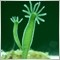• Get access

# I have open orders of currency pairs A, B, and C. Is there a way to get the expert to recognize that there is no open order of currency pair D?37

Hi, say I have open orders of currency pairs A, B, and C. Is there a way to get the expert to recognize that there is no open order of currency pair D, and given the right conditions, to then open one? Or to see that there already is an trade open for currency par A and not open one? I'm wanting to have this expert running on 4 different currency pairs but I only want one open trade at a time per currency pair. How do I do this? I've been thinking about it a while and I'm stuck.

thanks.77

There are a few ways to do it. The easiest might be to use the code in the moving average sample from MQ that came with MT4. What it does is calculate current orders in this function:

```//+------------------------------------------------------------------+
//| Calculate open positions                                         |
//+------------------------------------------------------------------+
int CalculateCurrentOrders(string symbol)
{
//----
for(int i=0;i<OrdersTotal();i++)
{
if(OrderSymbol()==Symbol() && OrderMagicNumber()==MAGICMA)
{
if(OrderType()==OP_SELL) sells++;
}
}
//---- return orders volume
else       return(-sells);
}```

And then in the start function, it only will seek to open an order if it doesn't already have one open:

```   if(CalculateCurrentOrders(Symbol())==0) CheckForOpen();
else                                    CheckForClose();```

Those are at least good pieces to start with. You could adjust it so instead of counting orders, you could just return true/false if there are any orders, and then make that function call a condition of the EA's open order test. Pretty similar to this one, very slightly different.289

I've made that with a little more code:

```void GbpUsdBuy() {
bool
result,
GbpUsdopen;
double
GbpUsdBid=MarketInfo("GBPUSD",MODE_BID),
GbpUsdPoint=MarketInfo("GBPUSD",MODE_POINT);
for(int GbpUsd=0;GbpUsd<OrdersTotal();GbpUsd++) {
if(OrderMagicNumber()!=Number || OrderSymbol()!="GBPUSD") continue;
if(GbpUsdopen==0)
if(result!=TRUE) Print("LastError = ",GetLastError());return;if(result==135) RefreshRates();}}

void GbpUsdSell() {
bool
result,
GbpUsdopen;
double
GbpUsdBid=MarketInfo("GBPUSD",MODE_BID),
GbpUsdPoint=MarketInfo("GBPUSD",MODE_POINT);
for(int GbpUsd=0;GbpUsd<OrdersTotal();GbpUsd++) {
if(OrderMagicNumber()!=Number || OrderSymbol()!="GBPUSD") continue;
if(OrderType()==OP_SELL) GbpUsdopen=1;}
if(GbpUsdopen==0)
if(result!=TRUE) Print("LastError = ",GetLastError());return;if(result==135) RefreshRates();}}

bool
result,
EurUsdopen;
double
EurUsdBid=MarketInfo("EURUSD",MODE_BID),
EurUsdPoint=MarketInfo("EURUSD",MODE_POINT);
for(int EurUsd=0;EurUsd<OrdersTotal();EurUsd++) {
if(OrderMagicNumber()!=Number || OrderSymbol()!="EURUSD") continue;
if(EurUsdopen==0)
if(result!=TRUE) Print("LastError = ",GetLastError());return;if(result==135) RefreshRates();}}

void EurUsdSell() {
bool
result,
EurUsdopen;
double
EurUsdBid=MarketInfo("EURUSD",MODE_BID),
EurUsdPoint=MarketInfo("EURUSD",MODE_POINT);
for(int EurUsd=0;EurUsd<OrdersTotal();EurUsd++) {
if(OrderMagicNumber()!=Number || OrderSymbol()!="EURUSD") continue;
if(OrderType()==OP_SELL) EurUsdopen=1;}
if(EurUsdopen==0)
if(result!=TRUE) Print("LastError = ",GetLastError());return;if(result==135) RefreshRates();}}```37

I ended up getting the output I was looking for with just this:

``` for(i=0;i<total;i++)
{
{
if(OrderSymbol()==Symbol())
{
sym++;
}
if(OrderSymbol()!=Symbol())
{
tok++;
}
}
}```

then if sym<1, I open an order up for the currency pair.